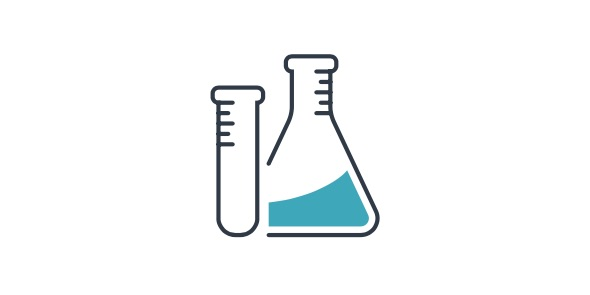# 7th Grade Science Chapter 1 Test-matter

36 Questions | Total Attempts: 1709Settings• 1.
Which of these is NOT matter?
• A.

A cloud

• B.

• C.

Sunshine

• D.

The sun

• 2.
You accidentally break your pencil in half. This is an example of:
• A.

A physical change

• B.

A chemical change

• C.

Density

• D.

Weight

• 3.
Which of the following statements about density is TRUE?
• A.

Density depends on mass and volume

• B.

Density is weight per unit volume

• C.

Density is measured in millimeters

• D.

Density is a chemical property

• 4.
Inertia increases as _____________ increases.
• A.

Time

• B.

Length

• C.

Mass

• D.

Volume

• 5.
An amount of vinegar would be expressed in ______________ for scientific experiments.
• A.

Centimeters or meters

• B.

Grams or milligrams

• C.

Liters or milliliters

• D.

Ounces or gallons

• 6.
During physical changes, matter always retains its:
• A.

Size

• B.

Identity (same type of particles)

• C.

State

• D.

Texture

• 7.
One way to compare the densities of oil and water is to pur the liquids into a glass container and observe how they:
• A.

Change color

• B.

Evaporate quickly

• C.

Separate into layers

• D.

Create an odor

• 8.
The amount of space taken up by an object is knows as the objects:
• A.

Mass

• B.

Weight

• C.

Inertia

• D.

Volume

• 9.
When a canoe overturns, a pocket of air forms inside the canoe because air:
• A.

Has volume

• B.

Takes up space even though it's invisible

• C.

Is matter

• D.

All of the above

• 10.
To measure the volume of water correctly, read the scale on a graduated cylinder at the _______________ part of the meniscus.
• A.

Bottom

• B.

Outer

• C.

Top

• D.

None of the above

• 11.
Weight and ______________ are the same thing.
• A.

Mass

• B.

Volume

• C.

Inertia

• D.

None of the above

• 12.
If you know the volume of the container a gas is in, then you know the ____________ of the gas.
• A.

Mass

• B.

Volume

• C.

Weight

• D.

Inertia

• 13.
Over time, you can expect the amount of mass to change in a:
• A.

Bowling ball

• B.

Child

• C.

Table

• D.

Nail

• 14.
Gravity affects an objects:
• A.

Weight

• B.

Mass

• C.

Volume

• D.

Density

• 15.
Which of the following best describes weight?
• A.

A measure of the amount of matter in an object

• B.

The same as mass

• C.

Weight is different depending on where the object is in the Universe

• D.

Expressed in kilograms

• 16.
The weight of an object depends on:
• A.

The mass of the object

• B.

The mass of Earth

• C.

The distance between the object and Earth

• D.

All of the above

• 17.
Tom's 7-year-old brother is more difficult to pick up than his 2-year-old sister because:
• A.

His brother has more inertia

• B.

His sister has more inertia

• C.

Boys are always heavier than girls

• D.

His brother has less mass than his sister

• 18.
Which of the following is an example of a physical change:
• A.

A silver spoon tarnishing

• B.

A cake baking in an oven

• C.

A popsicle melting

• D.

A car rusting

• 19.
The melting of butter when it is left out in a warm room is an example of:
• A.

A physical change

• B.

A chemical change

• C.

A physical property

• D.

A chemical property

• 20.
Although the Statue of Liberty is made of copper (originally an orange-brown color), it is green because the copper has interacted with substances in the air to form new substances with different properties. This is an example of:
• A.

A physical change

• B.

A chemical change

• C.

A physical property

• D.

A chemical property

• 21.
Considering that gold is one of the densest substances on Earth, which would you rather carry around school in your backpack all day, a kilogram  of gold or a kilogram of feathers?
• A.

Feathers, because your backpack would feel lighter

• B.

Gold, because your backpack would feel lighter

• C.

Gold, because it would take up less space in your backpack

• D.

Feathers, because they would take up less space in your backpack

• 22.
Precious metals in catalytic converters on cars change harmful carbon monoxide exhaust fumes to harmless ones. This is an exmple of a ______________ change.
• A.

Physical

• B.

Chemical

• C.

Characteristic

• D.

Both (a) and (c)

• 23.
Color, odor, mass and volume are ________ of an object
• A.

Chemical properties

• B.

Physical properties

• C.

Stationary properties

• D.

Inertial properties

• 24.
Flammability, solubility, and reactivity are ____________ of a substance.
• A.

Chemical properties

• B.

Physical properties

• C.

Stationary properties

• D.

Gravitational properties

• 25.
Being able to burn wood is an example of wood's:
• A.

Soluble properties

• B.

Chemical properties

• C.

Physical properties

• D.

Ductile properties

Related TopicsBack to top